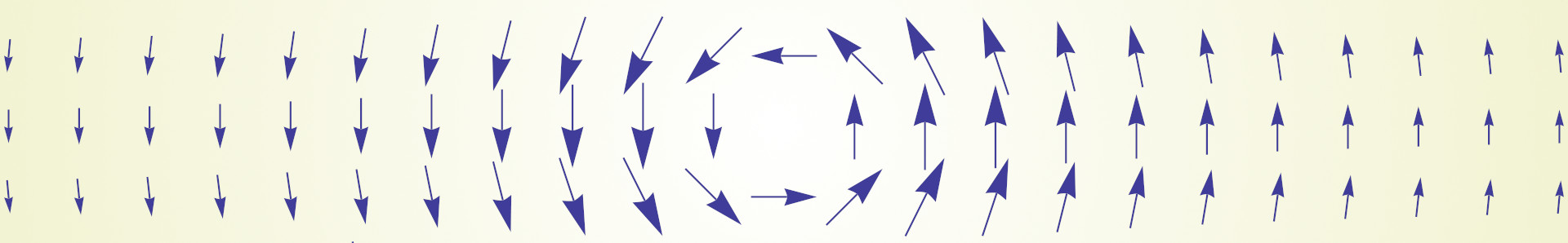Cheat sheet to differentiate expressions with the $$\nabla$$ operator to compute gradients of various functions.

### Usual operations

Sum rule, Product rule, sum rule, division rule, scalar rule ($$f$$ and $$g$$ both scalar functions $$g: \mathbb R^n \rightarrow \mathbb R$$, $$f: \mathbb R^n \rightarrow \mathbb R$$):

$$\begin{array}{lcl} \nabla [ f + g ] & = &\nabla f + \nabla g \\ \nabla [ f . g ] & = & \nabla f . g + f . \nabla g \\ \nabla \left [ \frac{f}{g} \right ] & = &\frac{\nabla f . g - f . \nabla g}{g^2} \\ \nabla [ \alpha . f ] & = & \alpha . \nabla f \end{array}$$

$$\nabla [ \| \vec {x} \| ] = \frac{ \vec {x}}{ \| \vec {x} \|}$$

$$\nabla [ \| \vec {x} \|^2 ] = 2 . \vec {x}$$

With $$M$$ a $$n \times n$$ matrix:

$$\nabla [ M \vec {x} ] = M$$

Likewise for rigid transformations (rotations $$M \in \mathbb R^{3 \times 3}$$ and translations $$\vec t \in \mathbb R^3$$):

$$\nabla [ M \vec {x} + \vec t ] = M$$

### Chain rules

With $$s: \mathbb R \rightarrow \mathbb R$$ univariate and $$f: \mathbb R^n \rightarrow \mathbb R$$ multivariate real valued the operation boils down to a uniform scale of the gradient:
$$\nabla \left [ s( f(\vec {x}) ) \right ] = s'( f(\vec {x}) ) \nabla f(\vec {x})$$

With $$m: \mathbb R^n \rightarrow \mathbb R^n$$ deformation map and $$f: \mathbb R^n \rightarrow \mathbb R$$ multivariate scalar function the operation boils down to transform the gradient with a matrix:

$$\nabla \left [ f( m(\vec {x}) ) \right ] = \mathbf{J}\left [ m(\vec {x}) \right ]^\mathsf{T} \nabla f(m(\vec {x}))$$

Where $$\mathbf{J}\left [ m(\vec {x}) \right ]^\mathsf{T}$$ denotes the transpose of the $$n \times n$$ Jacobian matrix.

### Related Jacobian rules

Jacobian of a linear function is the identity matrix $$I$$ times the slope $$a$$:
$$J[a \vec {x} + c] = a.I$$

### Related chain rule

A univariate differentiation can lead to the use of $$\nabla$$: with $$f: \mathbb R^n \rightarrow \mathbb R$$ multivariable scalar function and $$p: \mathbb R \rightarrow \mathbb R^n$$ a parametric function:

$$f'(g(x)) = \nabla f(g(x))^T . \vec {g'(x)}$$

In short, we do the dot product between the gradient of $$f$$ and the speed of $$g$$